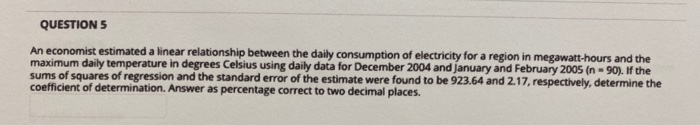# Question QUESTION 5 An economist estimated a linear relationship between the daily consumption of electricity for a region in megawatt-hours and the maximum daily temperature in degrees Celsius using daily data for December 2004 and January and February 2005 (n = 90). If the sums of squares of regression and the standard error of the estimate were found to be 923.64 and 2.17, respectively, determine the coefficient of determination. Answer as percentage correct to two decimal places.EYOA6G The Asker · Probability and StatisticsTranscribed Image Text: QUESTION 5 An economist estimated a linear relationship between the daily consumption of electricity for a region in megawatt-hours and the maximum daily temperature in degrees Celsius using daily data for December 2004 and January and February 2005 (n = 90). If the sums of squares of regression and the standard error of the estimate were found to be 923.64 and 2.17, respectively, determine the coefficient of determination. Answer as percentage correct to two decimal places.
More
Transcribed Image Text: QUESTION 5 An economist estimated a linear relationship between the daily consumption of electricity for a region in megawatt-hours and the maximum daily temperature in degrees Celsius using daily data for December 2004 and January and February 2005 (n = 90). If the sums of squares of regression and the standard error of the estimate were found to be 923.64 and 2.17, respectively, determine the coefficient of determination. Answer as percentage correct to two decimal places.